# Cost of Common Equity with and without Flotation

The Evanec Company's next expected dividend, D1, is \$3.18; its growth rate is 6%; and its common stock now sells for \$36. New stock (external equity) can be sold tonet \$32.40 a share.

What is Evanec's percentage flotation cost, F?

a.Cost of retained earnings = (D1/Current price)+Growth rate

=(\$3.18 / \$36 )+0.06

= 0.0883+ 0.06

= 0.1483

=14.83%

b.% flotation cost= (Current price - Net price)/Current price

=(36 – 32.40)/ 36

=10%

c. Cost of new common stock= (D1/Net price) + Growth rate

(3.18/32.40) + 0.06

= 0.0981 + 0.06

= 0.1581

=15.81 %

[REMOVED]

#### Earn Coin

Coins can be redeemed for fabulous gifts.

Similar Homework Help Questions
• ### cost of common equity with and ...

The Evanec Company's next expected dividend, D1, is \$3.18; its growth rate is 6%, and its common stock now sells for \$36.00. New stock (external equity) can besold to net \$32.40 per share.a) What is Evanec's cost of retained earning, rs ?b) What is Evanec's percentage flotation cost, F ?c) What is Evanec's cost of new common stock, re ?

• ### 7. Problem 10.07 (Cost of Common Equity with and thout Flotation) eBook The Evanec Company's next...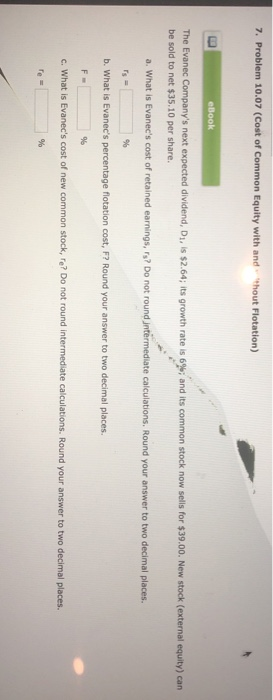7. Problem 10.07 (Cost of Common Equity with and thout Flotation) eBook The Evanec Company's next expected dividend, Di, is \$2.64; its growth rate is 6%; and its common stock now sells for \$39.00. New stock (external equity) can be sold to net \$35.10 per share. a. What is Evanec's cost of retained earnings, rs? Do not round jntermediate calculations. Round your answer to two decimal places. rs b. What is Evanec's percentage flotation cost, F7 Round your answer to...

• ### The Evanec Company's next expected

The Evanec Company's next expected dividend, D is \$3.18; its growth rate is 6 percent; and its common stock new sells for\$36. New stock (external equity) can be soldto net \$32.40per share.A. What is Evanec's cost for retained earnings, r?B. What is Evanec's percentage flotation cost, F?C. What is Evanec's cost of new common stock,re?

• ### The Evanec Company's next expected dividend, D1, is \$3.44; its growth rate is 5%; and its...

The Evanec Company's next expected dividend, D1, is \$3.44; its growth rate is 5%; and its common stock now sells for \$30.00. New stock (external equity) can be sold to net \$27.00 per share. What is Evanec's cost of retained earnings, rs? Do not round intermediate calculations. Round your answer to two decimal places. rs =   % What is Evanec's percentage flotation cost, F? Round your answer to two decimal places. F =   % What is Evanec's cost of new common stock,...

• ### The Evanec Company's next expected dividend, D1, is \$2.92; its growth rate is 4%; and its...

The Evanec Company's next expected dividend, D1, is \$2.92; its growth rate is 4%; and its common stock now sells for \$37.00. New stock (external equity) can be sold to net \$33.30 per share. What is Evanec's cost of retained earnings, rs? Do not round intermediate calculations. Round your answer to two decimal places. rs =   % What is Evanec's percentage flotation cost, F? Round your answer to two decimal places. F =   % What is Evanec's cost of new common stock,...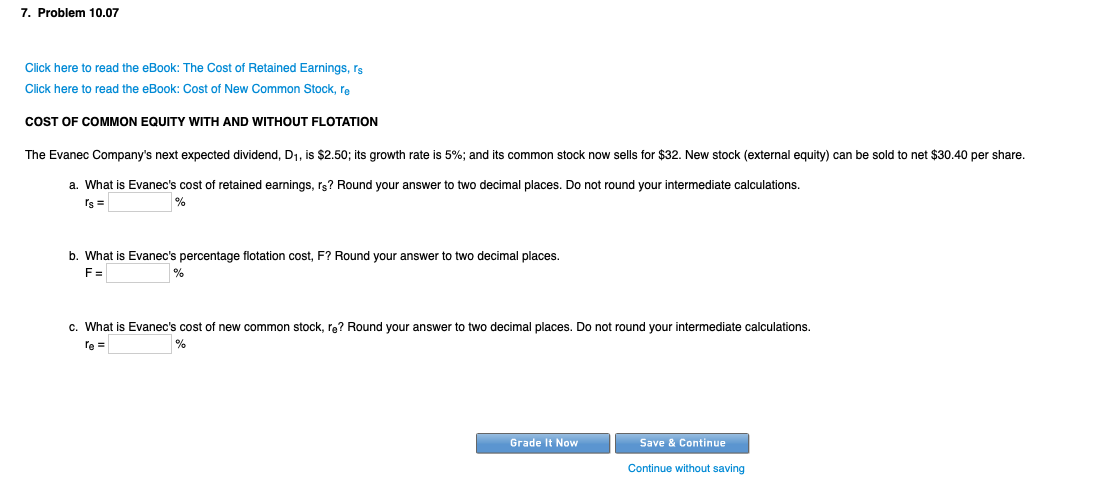7. Problem 10.07 Click here to read the eBook: The Cost of Retained Earnings, rs Click here to read the eBook: Cost of New Common Stock, le COST OF COMMON EQUITY WITH AND WITHOUT FLOTATION The Evanec Company's next expected dividend, D1, is \$2.50; its growth rate is 5%; and its common stock now sells for \$32. New stock (external equity) can be sold to net \$30.40 per share. a. What is Evanec's cost of retained earnings, rs? Round your...

• ### The Evanec Company's next expected dividend, D1, is \$2.76; its growth rate is 4%; and its...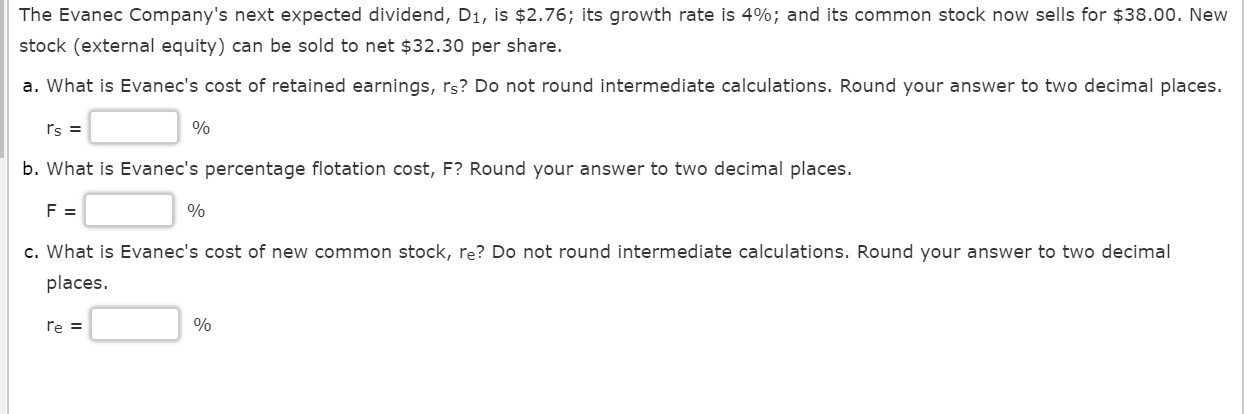The Evanec Company's next expected dividend, D1, is \$2.76; its growth rate is 4%; and its common stock now sells for \$38.00. New stock (external equity) can be sold to net \$32.30 per share. a. What is Evanec's cost of retained earnings, rs? Do not round intermediate calculations. Round your answer to two decimal places. Is = % b. What is Evanec's percentage flotation cost, F? Round your answer to two decimal places. F = % c. What is Evanec's...

• ### eBook The Evanec Company's next expected dividend, D1, is \$3.06; its growth rate is 6%; and...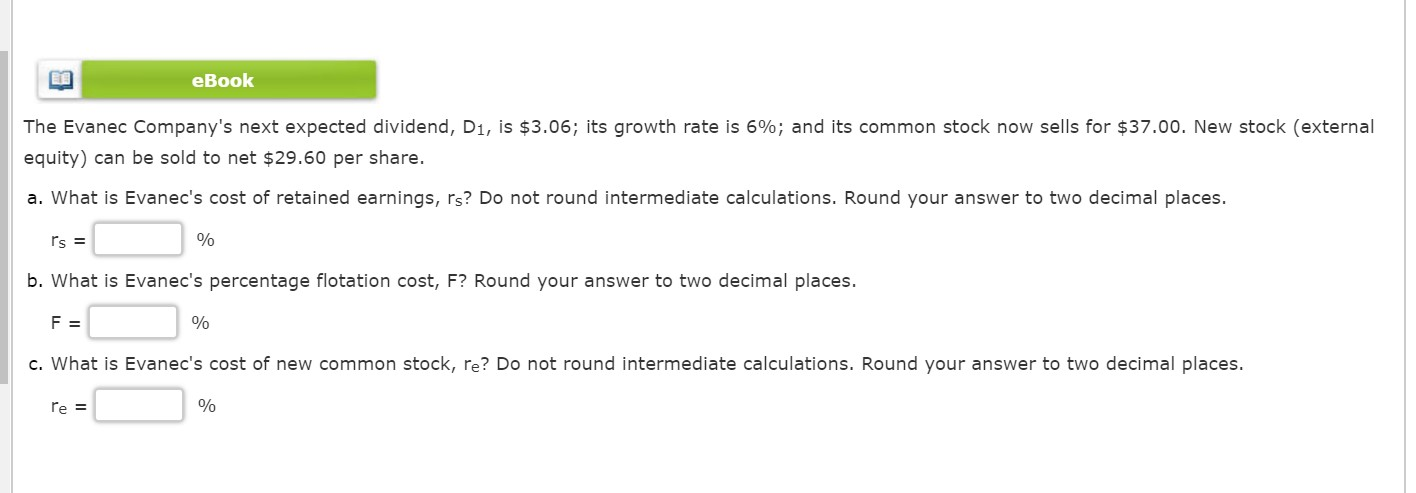eBook The Evanec Company's next expected dividend, D1, is \$3.06; its growth rate is 6%; and its common stock now sells for \$37.00. New stock (external equity) can be sold to net \$29.60 per share. a. What is Evanec's cost of retained earnings, rs? Do not round intermediate calculations. Round your answer to two decimal places. rs = b. What is Evanec's percentage flotation cost, F? Round your answer to two decimal places. F = 이이 c. What is Evanec's...

• ### The Evanec Company's next expected dividend, D1, is \$2.91; its growth rate is 6%; and its...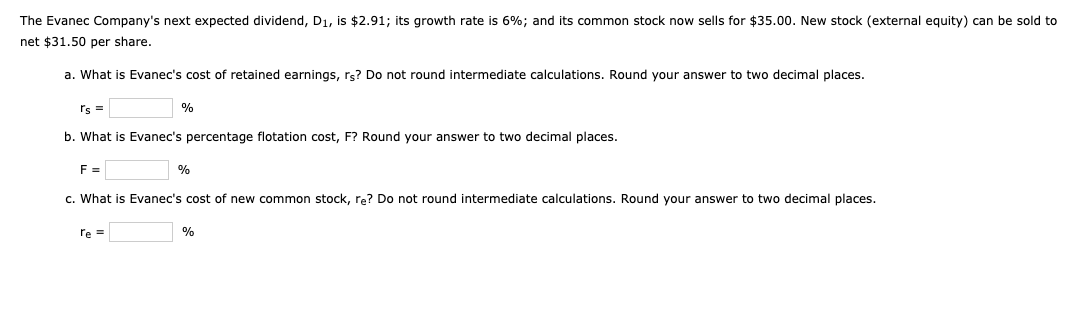The Evanec Company's next expected dividend, D1, is \$2.91; its growth rate is 6%; and its common stock now sells for \$35.00. New stock (external equity) can be sold to net \$31.50 per share. a. What is Evanec's cost of retained earnings, rs? Do not round intermediate calculations. Round your answer to two decimal places. Is = % b. What is Evanec's percentage flotation cost, F? Round your answer to two decimal places. F= % c. What is Evanec's cost...

• ### The Evanec Company's next expected dividend, D1, is \$2.86; its growth rate is 7%; and its...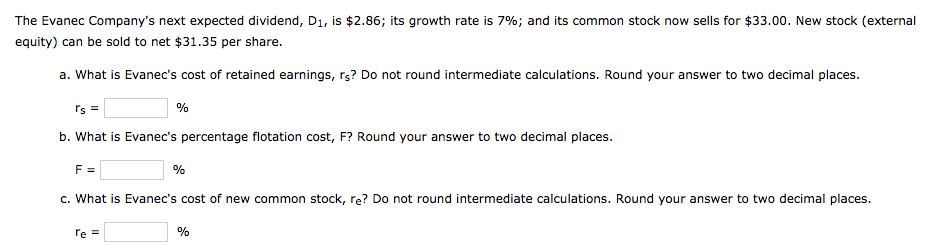The Evanec Company's next expected dividend, D1, is \$2.86; its growth rate is 7%; and its common stock now sells for \$33.00. New stock (external equity) can be sold to net \$31.35 per share. a. What is Evanec's cost of retained earnings, rs? Do not round intermediate calculations. Round your answer to two decimal places. Is = b. What is Evanec's percentage flotation cost, F? Round your answer to two decimal places. F= % c. What is Evanec's cost of...